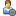###Show Posts

This section allows you to view all posts made by this member. Note that you can only see posts made in areas you currently have access to.

### Topics - penguins_demise

Pages: 
1
##### Flat Earth Debate / The seasons and angular momentum
« on: October 08, 2011, 05:03:22 PM »
In the FAQ, the seasons on a flat earth are described as being caused by a variation in the radius of the sun's motion. This, however, would violate conservation of angular momentum. The angular momentum of the sun would be:

L = I w = mr^2 w, where m is the mass of the sun, r is the radius of rotation and w is the angular velocity.

The angular velocity remains the same, as the length of each day remains at 24 hours. Hence, as r decreases, the angular momentum would decrease.

So, my question is, how do you reconcile this model of the seasons with conservation of angular momentum?

Pages: